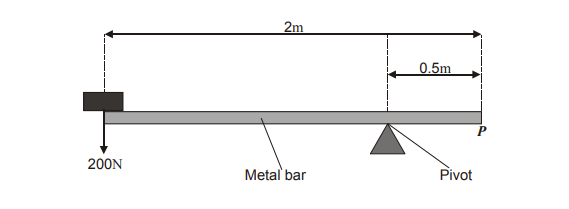# IMAT 2011 Q71 [Forces]

A uniform bar of length 2.0 m and weight 1000 N has its centre of gravity at its centre. The bar is pivoted in the position shown, and supports a weight of 200 N in the position shown in the diagram.What weight is needed at position P to balance the bar?

A. 600N
B. 800N
C. 1000N
D. 1600N
E. 1800N

@AriHoresh

For a uniform bar to be “stable” or in equilibrium, the Clockwise Moment must equate the Anti-Clockwise moment around the pivot.

A moment (M) in Newton meters is equal to the product of the Force (F) in Newtons and the perpendicular distance from the pivot (d) in meters.

M=Fd

With the provided values we are able to deduce the Anti-clockwise moment.

However beforehand, we must look at any additional information that may come into play.

Here, this information, or trick per say, is “A uniform bar of length 2.0 m and weight 1000 N has its centre of gravity at its centre”.

This means that for the Anti-clockwise moment, we must calculate 2 sets of values and add them to give us the final Anti-clockwise moment-

So it will look something like this:

M_{A1}= F \times d= 200N \times (2m - 0.5 m) = 200N \times 1.5 = 300 Nm

M_{A2} = F \times d = 1000N \times 0.5m = 500 Nm

Since the weight of the bar acts on it’s center of gravity at the centre of the bar (1m), the distance from the pivot will be 0.5m

M_{AT}= M_{A1} + M_{A2} = 300 Nm + 500 Nm = 800 Nm

Now, we proceed to look at the Clockwise Moment-

M_{C}= F \times d = 0.5F

We learn that we need to find this force or weight, F.

We can use the concept of Anti-clockwise moment= Clockwise Moment to find it:

M_{AT}= M_{C},

where M_{AT}= 800 Nm and M_{C}= 0.5F

So we can say

0.5F= 800 Nm

F= {\frac {800 Nm}{0.5 m}}= 1600 N

Which makes the weight needed at Position P to balance the bar 1600 N or choice (D).